# Other types of polygon

By Martin McBride, 2022-10-21
Tags: regular polygon convex polygon concave polygon complex polygon equilateral polygon equiangular polygon star polygon
Categories: gcse geometrySo far we have looked only looked at convex polygons, but there are actually 3 types:

• Convex polygons, where every interior angle is less than 180 degrees. In other words, none of the corners of the bend back into the shape. Most polygons we meet are convex.
• Concave polygons, where some interior angles are greater than 180 degrees, so some lines band back into the shape. An example of that would be an L-shaped polygon.
• Complex polygons, where some sides of the polygon cross over each other. A well-known example of this is a pentagram.

We will also look at:

• Equilateral polygons, where all the sides are equal but the angles might not be.
• Equiangular polygons, where all the angles are equal but the sides might not be.
• Star polygons, which can have equal sides and equal angles but are not conventional regular polygons.

This will show that we have to be careful how we define a regular polygon.

## Convex and concave polygons

A convex polygon is a polygon where none of the corners point inwards, and all the interior angles are less than 180 degrees. Here is an example of a convex and concave pentagon: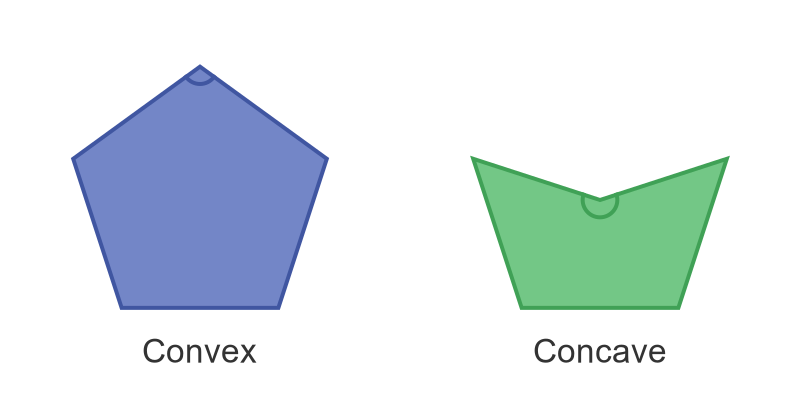As the diagram shows, in the convex polygon, all the corners point outwards from the centre of the shape. In the concave polygon, one or more of the corners points inwards towards the centre. In the example, only one corner points inwards.

Also in the convex case, all the interior angles are less than 180 degrees. In a convex polygon, one or more interior angles are greater than 180 degrees. Again the example has one angle that is greater than 180 degrees, the angle is marked on the diagram.

These two conditions are equivalent - if a shape has one or more inward-pointing corners then it will have one or more interior angles greater than 180 degrees, and vice versa.

## Convex hull of a polygon

Imagine we took a loop of string, place it around a polygon, the pulled it tight. This loop would tightly contain the polygon, like this: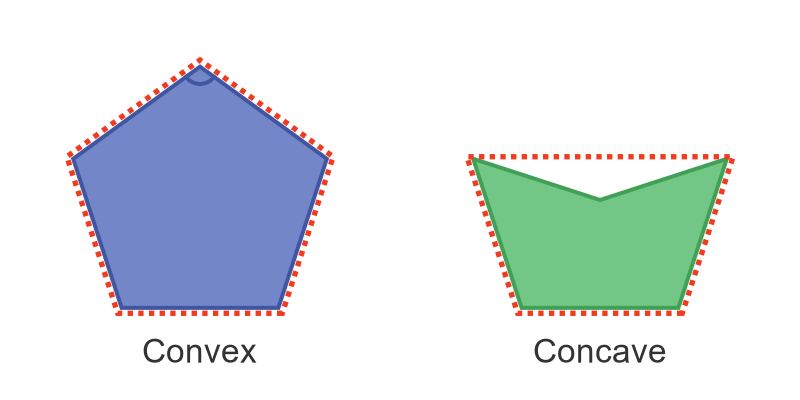The red, dotted boundary shows the convex hull of each shape.

The convex hull is the smallest convex polygon that contains all the vertices of the polygon.

For a convex polygon, the convex hull is exactly the same size and shape as the polygon itself.

For a concave polygon, the convex hull is a different shape from the polygon. The convex hull misses any inwards pointing vertices. In this case, the convex hull of the pentagon is a quadrilateral.

## Complex polygons

A complex polygon is a polygon that intersects itself (in other words, some of its sides cross). Here is an example of a regular heptagon and a complex heptagon: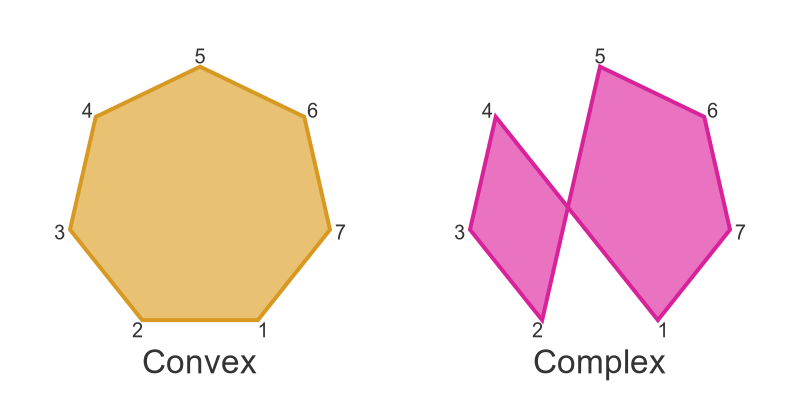In this example, both shapes have the same vertices, but the sides connect the vertices in a different order. In the convex polygon, the vertices are joined in the order 1 to 7. In the complex polygon, they are joined in the order (1, 4, 3, 2, 5, 6, 7).

Vertices 1 and 4 are joined by a single line. Vertices 2 and 5 are joined by a single line. Those lines cross, but each one is just a single line. There are 7 lines altogether.

Usually, in geometry, we assume that we are dealing with simple polygons (either convex or concave). Some of the rules that apply to simple polygons don't apply to complex polygons. For example, the sum of the exterior angles of a convex polygon is 360 degrees, but that is not true of a complex polygon. Also, with a complex polygon, it can be more difficult to determine if a particular point is inside or outside the polygon. This is described more in the section on star polygons below.

## Equilateral polygons

One feature of regular polygons is that all their sides are the same length. We say that regular polygons are equilateral (which just means all the sides are the same length). However, a shape can be equilateral but not regular. Here are some examples: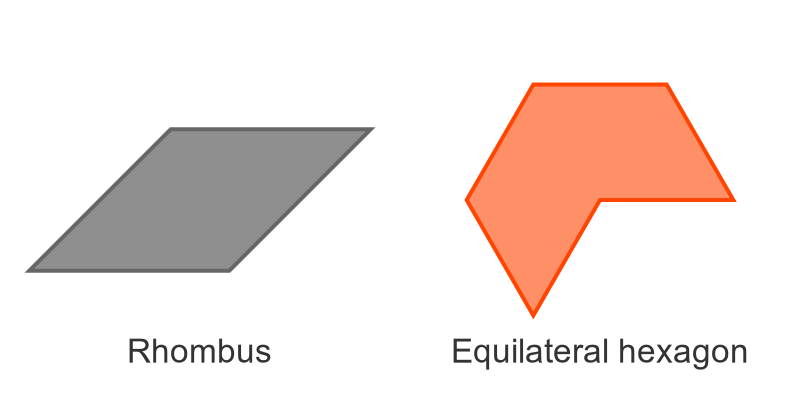The left-hand shape is a rhombus. A rhombus is just a "pushed-over" square. All four sides have the same length, but the angles aren't all the same so it is not a regular shape.

The right-hand shape is a hexagon. This is similar to a regular hexagon except that one corner is pushed in. Again all the sides are the same length but one angle is different so it is not a regular shape.

## Equiangular polygons

Another feature of regular polygons is that all their interior angles are equal. We say that regular polygons are equiangular. Again, a shape can be equiangular but not regular. Here are some examples:The left-hand shape is a rectangle. A rectangle has 4 right angles, like a square, but its sides aren't all the same length so it isn't a regular shape.

The right-hand shape is a hexagon. This is similar to a regular hexagon except that top and bottom horizontal sides have been stretched. This doesn't change any angles but it means that those two sides are not the same length as the others, so once again the shape is not regular.

## Star polygons

A star polygon is a special type of complex polygon. This diagram shows a regular pentagon and a star pentagon that has the same vertices: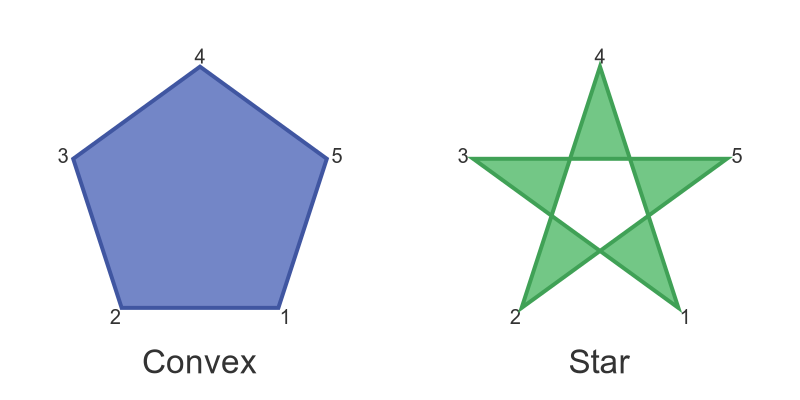Although the star pentagon shares the same vertices as the regular pentagon, it is a different shape because it joins the vertices in a different order. The thing that makes a star polygon special (compared to any other complex polygon) is that the order of the vertices follows a strict pattern.

In this case, each vertex is joined to the next-but-one vertex. Vertex 1 is joined to 3, 3 is joined to 5, 5 to 2, 2 to 4, and then back to 1 again.

A 5 sided star polygon is called a pentagram rather than a pentagon. Other stars have similar names, by replacing the "gon" with "gram", so a 6 side star is called a hexagram, and so on.

Since this star pattern is based on the vertices of a regular pentagon, this means that all the lines in the star are the same length, and all the internal angles are equal.

We call this shape a regular star polygon. This is different to a regular polygon.

Notice that the small upside-down pentagon in the centre of the star is not considered to be inside the polygon. To see why, look at this diagram: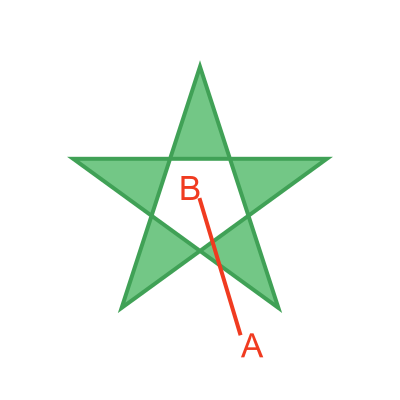A is definitely outside the shape. But to get from A to B we must:

• First cross the boundary of the shape, into the green triangular area. We would then be inside the shape.
• Then cross the boundary of the shape, again, into the central pentagonal area. Since we were previously inside the shape, and then crossed the shpe boundary again, we must now be outside the shape. So the inner pentagon is outside the shape.

## A new definition of regular polygons

Now we have learned about several other types of polygons, we need a better definition of a regular polygon. A regular polygon is a polygon that has:

• All its sides of equal length and
• All its internal angles equal and
• Is convex.